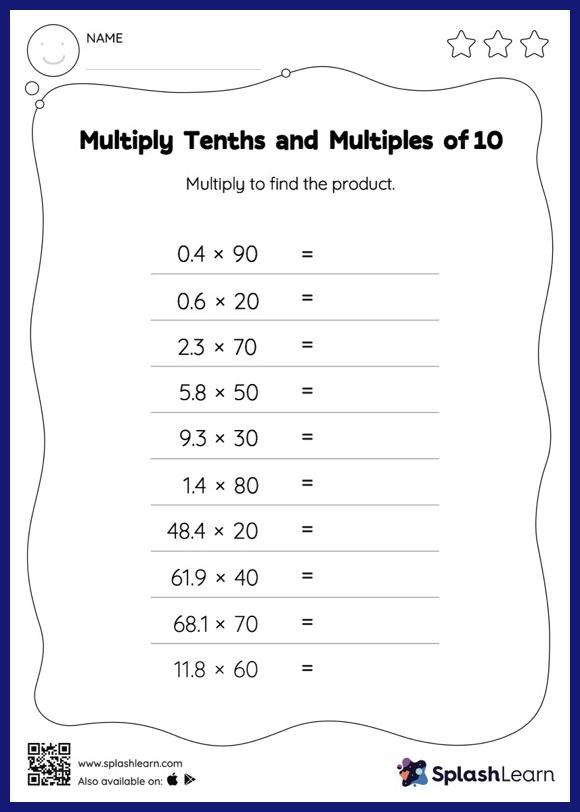# Multiply Tenths and Multiples of 10: Horizontal Multiplication Worksheet

Home > Multiply Tenths and Multiples of 10: Horizontal MultiplicationMultiply tenths and multiples of 10 worksheet helps students develop proficiency with multiplication. Students know that when multiplying a decimal by a number ending in zeros, the decimal point is first ignored and then placed in the product as many places from the right as the number of decimal digits in the original number. They practice this idea thoroughly in multiply tenths and multiples of 10 worksheet.